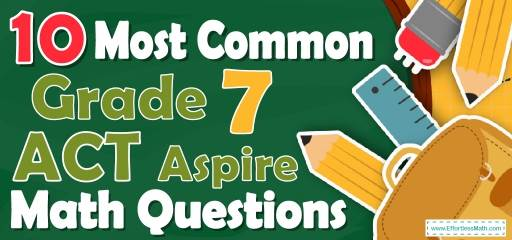# 10 Most Common 7th Grade ACT Aspire Math QuestionsEveryone knows the importance of using practice questions to pass the 7th Grade ACT Aspire Math test. Using 7th Grade ACT Aspire Math practice questions helps students to know what questions they are likely to face in the test and how they should study. 7th Grade Students also need to know which parts Are more important and which parts are less important. The use of 7th Grade ACT Aspire Math practice test questions addresses these needs. Therefore, in this article, we have provided the 10 most common 7th Grade ACT Aspire Math questions for your students. The answers are also given in detail at the end of the article.

Make sure to follow some of the related links at the bottom of this post to get a better idea of what kind of mathematics questions students need to practice.

## 10 Sample 7th Grade ACT Aspire Math Practice Questions

1- What is the median of these numbers? $$2, 28, 28, 19, 67, 44, 35$$

A. $$19$$

B. $$28$$

C. $$44$$

D. $$35$$

2- Last week $$24,000$$ fans attended a football match. This week three times as many bought tickets, but one-sixth of them canceled their tickets. How many are attending this week?

A. $$48000$$

B. $$54000$$

C. $$60000$$

D. $$72000$$

3- The following trapezoids are similar. What is the value of $$x$$?

A. $$7$$

B. $$8$$

C. $$18$$

D. $$45$$

4- If $$x=- 8$$, which equation is true?

A. $$x(2x-4)=120$$

B. $$8 (4-x)=96$$

C.$$2 (4x+6)=79$$

D. $$6x-2=-46$$

5- In a bag of small balls $$\frac{1}{3}$$ are black, $$\frac{1}{6}$$ are white, $$\frac{1}{4}$$ are red and the remaining $$12$$ blue. How many balls are white?

A. $$8$$

B. $$12$$

C. $$16$$

D. $$24$$

6- A boat sails $$40$$ miles south and then $$30$$ miles east. How far is the boat from its start point?

A. $$45$$

B. $$50$$

C. $$60$$

D. $$70$$

7- Sophia purchased a sofa for $$530.40$$. The sofa is regularly priced at $$624$$. What was the percent discount Sophia received on the sofa?

A. $$12\%$$

B. $$15\%$$

C. $$20\%$$

D. $$25\%$$

8- The score of Emma was half as that of Ava and the score of Mia was twice that of Ava. If the score of Mia was $$60$$, what is the score of Emma?

A. $$12$$

B. $$15$$

C. $$20$$

D. $$30$$

9- A bag contains $$18$$ balls: two green, five black, eight blue, a brown, a red, and one white. If $$17$$ balls are removed from the bag at random, what is the probability that a brown ball has been removed?

A. $$\frac{1}{9}$$

B. $$\frac{1}{6}$$

C. $$\frac{16}{17}$$

D. $$\frac{17}{18}$$

10- A rope weighs $$600$$ grams per meter in length. What is the weight in kilograms of $$12.2$$ meters of this rope? $$(1 kilograms = 1000 grams)$$

A. $$0.0732$$

B. $$0.732$$

C. $$7.32$$

D. $$7320$$

## Best 7th Grade ACT Aspire Math Prep Resource for 2022

1- B
Write the numbers in order:
$$2, 19, 28, 28, 35, 44, 67$$
Since we have $$7$$ numbers $$(7$$ is odd), then the median is the number in the middle, which is $$28$$.

2- C
Three times $$24,000$$ is $$72,000$$. One-sixth of them canceled their tickets.
One sixth of $$72,000$$ equals $$12,000$$ $$(\frac{1}{6}) × 72000 = 12000$$.
$$60,000$$ $$(72000 – 12000 = 60000)$$ fans are attending this week

3- A
It’s needed to have a ratio to find value of $$x$$.
$$\frac{45}{40}=\frac{2x+4}{16}⇒ 40(2x+4)=45×16 ⇒ x=7$$

4- C
$$8 (4-(-8))=96$$

5- A
$$\frac{1}{3}x + \frac{1}{6}x + \frac{1}{4}x + 12= x$$
$$(\frac{1}{3} + \frac{1}{6} + \frac{1}{4}) x+ 12= x$$
$$(\frac{9}{12})x+ 12 = x$$
$$x = 48$$
In a bag of small balls $$\frac{1}{6}$$ are white then: $$\frac{48}{6} = 8$$

6- B
Use the information provided in the question to draw the shape.
Use Pythagorean Theorem: $$a^2 + b^2 = c^2$$
$$40^2 + 30^2 = c^2 ⇒ 1600 + 900 = c^2 ⇒ 2500 = c^2 ⇒ c = 50$$

7- B
The question is this: $$530.40$$ is what percent of $$624$$?
Use percent formula:
$$part = \frac{percent}{100}× whole$$
$$530.40= \frac{percent}{100}× 624 ⇒ 530.40 = \frac{percent ×624}{100}⇒53040 = percent ×624$$
$$⇒percent = \frac{53040}{624}= 85$$
$$530.40$$ is $$85 \%$$ of $$624$$. Therefore, the discount is: $$100\% – 85\% = 15\%$$

8- B
If the score of Mia was $$60$$, therefore the score of Ava is $$30$$. Since the score of Emma was half as that of Ava, therefore, the score of Emma is $$15$$.

9- D
If $$17$$ balls are removed from the bag at random, there will be one ball in the bag.
The probability of choosing a brown ball is $$1$$ out of $$18$$. Therefore, the probability of not choosing a brown ball is $$17$$ out of $$18$$ and the probability of having not a brown ball after removing $$17$$ balls is the same.

10- C
The weight of $$12.2$$ meters of this rope is: $$12.2 × 600 \space g = 7320 \space g$$
$$1\space kg = 1000 \space g$$ therefore,
$$7320 \space g ÷ 1000 = 7.32 \space kg$$

Looking for the best resource to help you succeed on the Grade 7 ACT Aspire Math test?

## The Best Books to Ace 7th Grade ACT Aspire Math Test

### What people say about "10 Most Common 7th Grade ACT Aspire Math Questions - Effortless Math: We Help Students Learn to LOVE Mathematics"?

No one replied yet.

X
26% OFF

Limited time only!

Save Over 26%

SAVE $5 It was$19.99 now it is \$14.99